# Credit Scorecard Validation Metrics

This example shows how to implement a probability of default (PD) model validation suite covering the techniques laid out in . The techniques in  apply to any PD model. In this example, you load the test data, set and compute some key quantities, and calculate some model validation metrics. The results are stored in a collection object. You use the `PDModel` data set, which consists of a pre-fitted MATLAB® credit scorecard object. You then compute various metrics and summarize them.

### Prepare Data

```modelDataFile = "PDModel.mat"; inputData = load(modelDataFile); ```

Set the response variable and default indicator.

```responseVar = "status"; outcomeIndicator = 1;```

Extract key data and precompute certain key quantities

```sc = inputData.sc; testData = sc.Data; scores = sc.score; defaultProbs = probdefault(sc); defaultIndicators = testData.(responseVar) == outcomeIndicator; ```

### Visualize Cumulative Accuracy Profile and Compute Accuracy Ratio

The cumulative accuracy profile (CAP) curve shows the proportion of defaulting names against the proportion of all names in the test set as the scores range from lower to higher values. The accuracy ratio (AR) measures how the model relates to hypothetical perfect and random models. An AR of 1 indicates the model is perfect, whereas an AR of 0 indicates the scoring model is no better than a random model.

Create a collection object for storing metrics.

`MH = mrm.data.validation.MetricsHandler;`

Calculate the CAP values and the AR. Plot the CAP curve.

```CAPMetric = mrm.data.validation.pd.CAPAccuracyRatio(defaultIndicators,scores); visualize(CAPMetric);```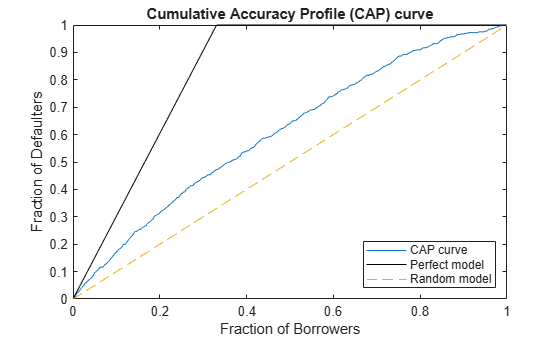Display the accuracy ratio and append the CAP values to the collection object.

`displayResult(CAPMetric);`
```Accuracy ratio is 0.3223 ```
`append(MH,CAPMetric);`

### Compute and Visualize Receiver Operating Characteristic

The receiver operating characteristic (ROC) curve shows the proportion of defaulting names against the proportion of non-defaulting names in the test set as the scores move from lower to higher values. The associated test metric is the area under the ROC curve (AUROC). An AUROC value of 1 indicates a perfect model.

Calculate the ROC values and plot the ROC curve.

```ROCMetric = mrm.data.validation.pd.AUROC(defaultIndicators,scores); visualize(ROCMetric);```Display the AUROC value and append the ROC values to the collection object.

`displayResult(ROCMetric);`
```Area under ROC curve is 0.6611 ```
`append(MH,ROCMetric);`

### Compute and Visualize Kolmogorov-Smirnov Statistic

Calculate and plot the Kolmogorov-Smirnov statistic, which indicates the maximal separation of defaulters from non-defaulters that the model achieves. The two plots in the visualization are the fractions of defaulters and non-defaulters in the test set. Reference  refers to these quantities as the hit rate and the false alarm rate, respectively.

```KSMetric = mrm.data.validation.pd.KSStatistic(defaultIndicators,scores); visualize(KSMetric);```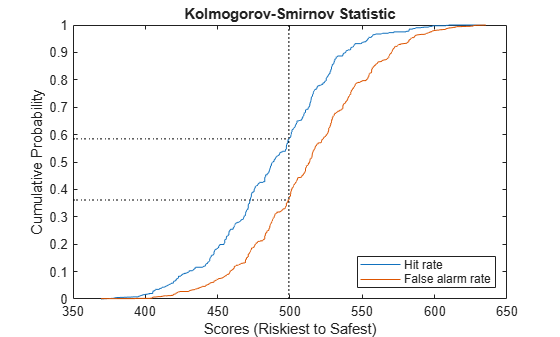Display the Kolmogorov-Smirnov statistic and append the values to the collection object.

`displayResult(KSMetric);`
```Kolmogorov-Smirnov statistic is 0.2232, at score 499.2 ```
`append(MH,KSMetric);`

### Compute and Visualize Pietra Index

The Pietra Index is twice the maximal area of a triangle that can be fit between the ROC curve and the diagonal. Multiplication by 2 ensures that the range of possible values for the index is [0, 1].

Calculate and plot the Pietra Index. Assume that the area under the ROC is convex. In this case, the Pietra Index matches the Kolmogorov-Smirnov statistic, indicated by the vertical dotted line in the plot.

```PietraMetric = mrm.data.validation.pd.PietraIndex(defaultIndicators,scores); visualize(PietraMetric);```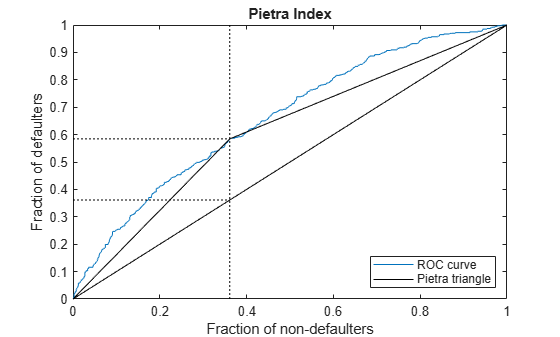Display the Pietra metric and append it to the collection object.

`displayResult(PietraMetric);`
```Pietra index is 0.2232 ```
`append(MH,PietraMetric);`

### Compute and Visualize Bayesian Error Rate

Define the error rate at a given score as this weighted sum:

$ER\left(s\right)={p}_{D}\left(1-HR\left(s\right)\right)+\left(1-{p}_{D}\right)FAR\left(s\right)$,

where ${p}_{D}$ is the total proportion of defaulters in the test set, $HR$ is the hit rate function, and $FAR$ is the false alarm rate function. The Bayesian error rate of the model is the minimum error rate in the test set. The error rate is sometimes calculated with equal weights of 0.5 instead of ${p}_{D}$ and $1-{p}_{D}$. In this case the Bayesian error rate is equal to $\frac{1}{2}\left(1-KS\right)$, where $KS$ is the Kolmogorov-Smirnov statistic.

Calculate and plot the Bayesian error rate.

```BERMetric = mrm.data.validation.pd.BayesianErrorRate(defaultIndicators,scores); visualize(BERMetric);```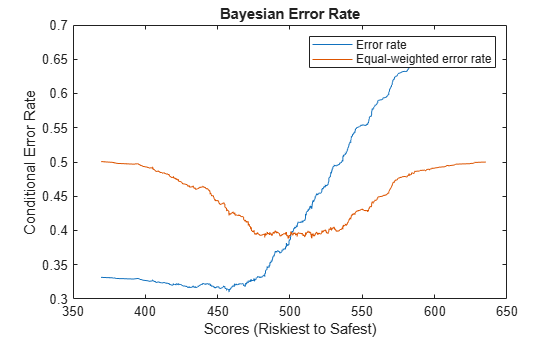Display the Bayesian error rate statistics in a table and append the values to a collection object.

`displayResult(BERMetric);`
``` Value Score _______ ______ Bayesian error rate 0.31 457.9 Equal-weighted Bayesian error rate 0.38838 499.18 ```
`append(MH,BERMetric);`

### Calculate Mann-Whitney Statistic

The Mann-Whitney statistic is a rank sum metric that you calculate for the defaulter and non-defaulter populations of the test set. This metric recovers the AUROC statistic. You can use the variance of the Mann-Whitney statistic to calculate confidence intervals for the AUROC statistic.

Calculate the Mann-Whitney statistic, display the result, and append it to the collection object.

```mwConfidenceLevel =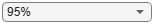0.95; MWMetric = mrm.data.validation.pd.MannWhitneyMetric(defaultIndicators,scores, ... mwConfidenceLevel); displayResult(MWMetric);```
```Mann-Whitney statistic 0.66113, with confidence interval (0.6606,0.66165) at level 0.95 ```
`append(MH,MWMetric);`

### Calculate Somers' D Value

To test the hypothesis that a low score correspond to a high probability of default, calculate the Somers' D between the sorted scores and the default indicators (flipped, to preserve the ordering, so that survival is denoted by 1 and default by 0).

```SDMetric = mrm.data.validation.pd.SomersDMetric(defaultIndicators,scores); displayResult(SDMetric);```
```Somers' D is 0.3223 ```
`append(MH,SDMetric)`

### Display Brier Score

The Brier score is the mean squared error of the probabilities of default that the model predicts. Calculate the Brier score also for the model that assigns the same probability to each name (equal to the fraction of defaulting names in the data set). Display the result and append it to the collection object.

```BrierMetric = mrm.data.validation.pd.BrierTestMetric(defaultIndicators,defaultProbs); displayResult(BrierMetric); ```
``` Value _______ Brier score 0.20541 Brier score for trivial model 0.22138 ```
`append(MH,BrierMetric);`

### Perform Binomial Test

The binomial test inspects each rating category separately and tests whether the number of realized defaults is plausible for the predicted probability of default.

Set the confidence level using the `q` variable. For example, to calculate the scores at which the number of observed defaults exceeds the expected defaults with 95% confidence, set `q to 0.95`. Plot the test results, highlighting the scores with an asterisk.

```binConfidenceLevel =0.95; BinMetric = mrm.data.validation.pd.BinomialTest(defaultIndicators,defaultProbs,scores,... binConfidenceLevel); visualize(BinMetric);```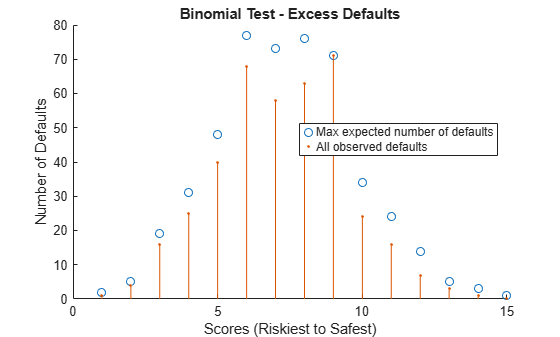Display the test results in a table and append the metric to the collection object.

`head(formatResult(BinMetric));`
``` Rating Category Observed Defaults Max Expected Number of Defaults _______________ _________________ _______________________________ 369.4 0 1 377.86 1 1 379.78 1 1 391.81 1 1 394.77 0 1 395.78 1 1 396.95 1 1 398.37 1 1 ```
`append(MH,BinMetric);`

### Compute Hosmer-Lemeshow Metric

The Hosmer-Lemeshow metric, also known as the chi-square test, compares the number of observed defaults for each ratings category with the expected number from the probability of default that the model predicts. Unlike the results of the binomial test, these measures are combined into a single quantity, which for large data sets converges to a ${\chi }_{k}^{2}$-distribution, where $k$ is the number of rating categories in the model. The test statistic is the $p$-value of this ${\chi }_{k}^{2}$-distribution.

```HLMetric = mrm.data.validation.pd.HosmerLemeshowMetric(defaultIndicators,defaultProbs,scores); displayResult(HLMetric);```
```Hosmer-Lemeshow p-value is 0.9177 ```
`append(MH,HLMetric);`

### Compute and Visualize Entropy Measures

The conditional information entropy ratio (CIER) measures the capability of the model to separate defaulters from non-defaulters. Unlike the other metrics you calculate in this example, the CIER does not depend on the ordering of the scores. A CIER value close to zero indicates good separation of defaulting names from surviving names.

Calculate and plot the CIER. The plot shows the cumulative sum of the entropies at each score weighted by the proportion of names with that score. Jumps indicate points at which the model struggles to differentiate between defaulters and non-defaulters.

```CIERMetric = mrm.data.validation.pd.EntropyMetric(defaultIndicators,scores); visualize(CIERMetric);```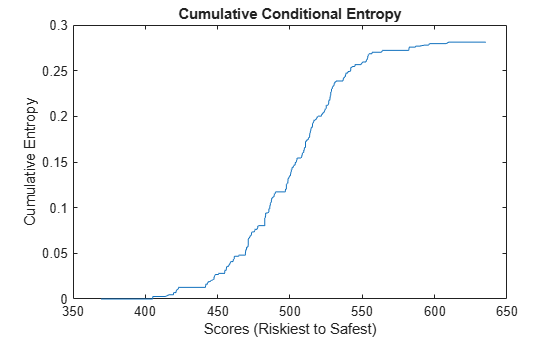Display the CIER and append the statistics to the collection object.

`displayResult(CIERMetric);`
```Conditional Information Entropy Ratio is 0.557 ```
`append(MH,CIERMetric);`

### Display Summary

Display a summary of the calculated statistics for the example model.

`disp(report(MH))`
``` Metric Value _______________________________________ ________ "Accuracy ratio" 0.32225 "Area under ROC curve" 0.66113 "Kolmogorov-Smirnov statistic" 0.22324 "Pietra index" 0.22324 "Bayesian error rate" 0.31 "Mann-Whitney u-test" 0.66113 "Somers' D" 0.32225 "Brier score" 0.20541 "Binomial test failure rate" 0.087667 "Hosmer-Lemeshow p-value" 0.91772 "Conditional Information Entropy Ratio" 0.55697 ```

### References

 Basel Committee on Banking Supervision, Working Paper 14. "Studies on the Validation of Internal Rating Systems (revised)." Bank for International Settlements. https://www.bis.org/publ/bcbs_wp14.htm.